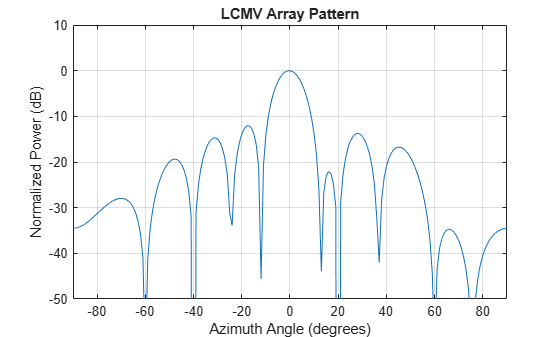# lcmvweights

Narrowband linearly constrained minimum variance (LCMV) beamformer weights

## Syntax

``wt = lcmvweights(constr,resp,cov)``

## Description

example

````wt = lcmvweights(constr,resp,cov)` returns narrowband linearly-constrained minimum variance (LCMV) beamformer weights, `wt`, for a phased array. When applied to the elements of the array, these weights steer the response of the array toward a specific arrival direction or set of directions. LCMV beamforming requires that the beamformer response to signals from a direction of interest are passed with specified gain and phase delay. However, power from interfering signals and noise from all other directions is minimized. Additional constraints may be imposed to specifically nullify output power coming from known directions. The constraints are contained in the matrix, `constr`. Each column of `constr` represents a separate constraint vector. The desired response to each constraint is contained in the response vector, `resp`. The argument `cov` is the sensor spatial covariance matrix. All elements in the sensor array are assumed to be isotropic.```

## Examples

collapse all

Construct a 10-element half-wavelength-spaced line array. Then, compute the LCMV weights for a desired arrival direction of 0 degrees azimuth. Impose three direction constraints : a null at -40 degrees, a unit desired response in the arrival direction 0 degrees, and another null at 20 degrees. The sensor spatial covariance matrix includes two signals arriving from -60 and 60 degrees and -10 dB isotropic white noise.

```N = 10; d = 0.5; elementPos = (0:N-1)*d; sv = steervec(elementPos,[-40 0 20]); resp = [0 1 0]'; Sn = sensorcov(elementPos,[-60 60],db2pow(-10));```

Compute the beamformer weights.

`w = lcmvweights(sv,resp,Sn);`

Plot the array pattern for the computed weights.

```vv = steervec(elementPos,[-90:90]); plot([-90:90],mag2db(abs(w'*vv))) grid on axis([-90,90,-50,10]); xlabel('Azimuth Angle (degrees)'); ylabel('Normalized Power (dB)'); title('LCMV Array Pattern');```The above figure shows that maximum gain is attained at 0 degrees as expected. In addition, the constraints impose nulls at -40 and 20 degrees and these can be seen in the plot. The nulls at -60 and 60 degrees arise from the fundamental property of the LCMV beamformer of suppressing the power contained in the two plane waves that contributed to the sensor spatial covariance matrix.

## Input Arguments

collapse all

Constraint matrix specified as a complex-valued, N-by-K, complex-valued matrix. In this matrix N represents the number of elements in the sensor array while K represents the number of constraints. Each column of the matrix specifies a constraint on the beamformer weights. The number of K must be less than or equal to N.

Example: [0, 0, 0; .1, .2, .3; 0,0,0]

Data Types: `double`
Complex Number Support: Yes

Desired response specified as complex-valued, K-by-1 column vector where K is the number of constraints. The value of each element in the vector is the desired response to the constraint specified in the corresponding column of `constr`.

Example: [45;0]

Data Types: `double`
Complex Number Support: Yes

Sensor spatial covariance matrix specified as a complex-valued, N-by-N matrix. In this matrix, N represents the number of sensor elements. The covariance matrix consists of the variances of the element data and the covariance between sensor elements. It contains contributions from all incoming signals and noise.

Example: [45;0]

Data Types: `double`
Complex Number Support: Yes

## Output Arguments

collapse all

Beamformer weights returned as an N-by-1, complex-valued vector. In this vector, N represents the number of elements in the array.

collapse all

### Linear-Constrained Minimum Variance Beamformers

The LCMV beamformer computes weights that minimize the total output power of an array but that are subject to some constraints (see Van Trees , p. 527). In order to steer the response of the array to a particular arrival direction, weights are chosen to produce unit gain when applied to the steering vector for that direction. This requirement can be thought of as a constraint on the weights. Additional constraints may be applied to nullify the array response to signals from other arrival directions such as those containing noise sources. Let (az1,el1),(az2,el2),...,(azK,elK) be the set of directions for which a constraint is to be imposed. Each direction has a corresponding steering vector, ${c}_{k}$, and the response of the array to that steering vector is given by ${c}_{k}^{H}w$. The transpose conjugate of a vector is denoted by the superscript symbol H. A constraint is imposed when a desired response is required when the beamformer weights act on a steering vector, ${c}_{k}$,

`${c}_{k}^{H}w={r}_{k}$`

This response could be specified as unity to allow the array to pass through the signal from a certain direction. It could be zero to nullify the response from that direction. All the constraints can be collected into a single matrix, C, and all the response into a single column vector, $R$. This allows the constraints to be represented together in matrix form

`${C}^{H}w=R$`

The LCMV beamformer chooses weights to minimize the total output power

`$P={w}^{H}Sw$`

subject to the above constraints. S denotes the sensor spatial correlation matrix. The solution to the power minimization is

`$w={S}^{-1}C{\left({C}^{H}{S}^{-1}C\right)}^{-1}R$`

and its derivation can be found in .

 Van Trees, H.L. Optimum Array Processing. New York, NY: Wiley-Interscience, 2002.

 Johnson, Don H. and D. Dudgeon. Array Signal Processing. Englewood Cliffs, NJ: Prentice Hall, 1993.

 Van Veen, B.D. and K. M. Buckley. “Beamforming: A versatile approach to spatial filtering”. IEEE ASSP Magazine, Vol. 5 No. 2 pp. 4–24.